Next: Occupation Number and Energy Up: Definitions of Number and Previous: Rescaled Fourier Transforms

### Conformal Coordinates

The equation of motion for a scalar field in an expanding universe is(5.10)

To define an adiabatic invariant occupation number for this field we need to switch to conformal variables in which this equation becomes a more standard oscillator equation. These variables are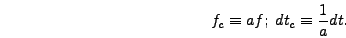(5.11)

Using conformal time and noting that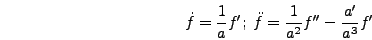(5.12)

the equation of motion becomes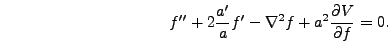(5.13)

Then switching to conformal field values note that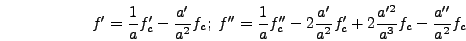(5.14)

and thus(5.15)

This equation can be approximated in Fourier space by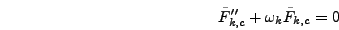(5.16)

where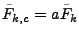and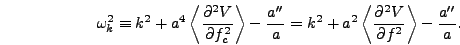(5.17)Next: Occupation Number and Energy Up: Definitions of Number and Previous: Rescaled Fourier Transforms